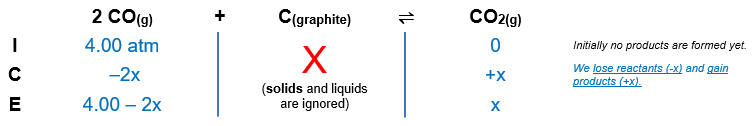# Problem: Given that Kp = 1.5 x 10-4 for the reaction 2 CO(g) &lt;=&gt; C(graphite) + CO2(g), what is the partial pressure of CO2(g) at equilibrium if initially 4.00 atm of CO(g) is in contact with graphite?a. 3.9952 atmb. 1670 atmc. 0.0083 atmd. 835 atme. 0.0024 atm

###### FREE Expert Solution

We’re being asked to calculate the partial pressure of CO2(g) at equilibrium if initially, 4.00 atm of CO(g) is in contact with graphite:

2 CO(g) C(graphite) + CO2(g);  Kp = 1.5 x 10-4

We're given the initial pressure of COs:

PCO = 4.00 atm

From this, we can construct an ICE table:The Kp expression for the reaction is:###### Problem Details

Given that Kp = 1.5 x 10-4 for the reaction 2 CO(g) <=> C(graphite) + CO2(g), what is the partial pressure of CO2(g) at equilibrium if initially 4.00 atm of CO(g) is in contact with graphite?

a. 3.9952 atm
b. 1670 atm
c. 0.0083 atm
d. 835 atm
e. 0.0024 atm